JEE  >  Single Correct MCQs: Definite Integrals & Application of Integrals

# Single Correct MCQs: Definite Integrals & Application of Integrals - Notes | Study All Types of Questions for JEE - JEE

 1 Crore+ students have signed up on EduRev. Have you?

Q.1.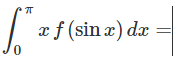(a)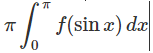(b)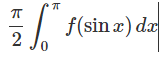(c)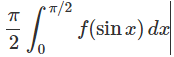(d) None of these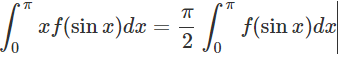Since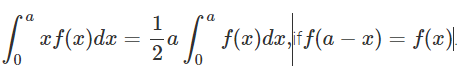Q.2. The area bounded by the curves y2 = 4x and y = x is equal to
(a) 1/3
(b) 8/3
(c) 35/6
(d) None of these

For the given curves, y2 = 4x and y = x, the intersection points are (0, 0) and (4, 4).

Therefore, the area bounded by the curves,

A =0∫4 [√(4x)-x] dx [since y2 = 4x, y = √(4x) ]

A = 2. 0∫4√x dx – 0∫4 x dx

Integrate the function and apply the limits, we get

A = (4/3)(8) – 8

A = (32-24)/3 = 8/3.

Hence, option (b) is the correct answer.

Q.3.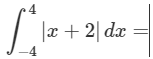(a) 50
(b) 24
(c) 20
(d) None of these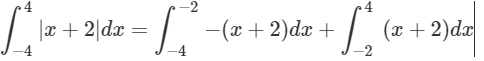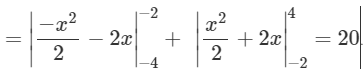Q.4. The area of the figure bounded by the curve y = logex, the x-axis and the straight line x = e is
(a) 5 - e
(b) 3 + e
(c) 1
(d) None of these

At, x= 1, y = loge (1) = 0
At, x = e, y = loge (e) = 1
Therefore, A = 1e logex dx
Using integration by parts,
A = [x loge x – x]1e
Now, apply the limits, we get
A = [e-e-0+1]
A = 1

Q.5.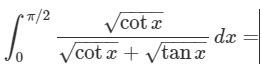(a) π|
(b) π/2|
(c) π/4|
(d) π/3|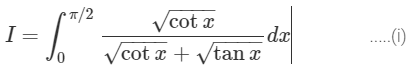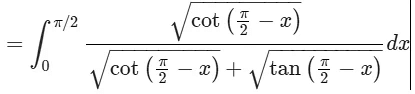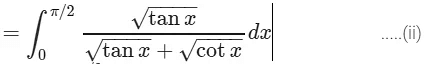Now adding (i) and (ii), we get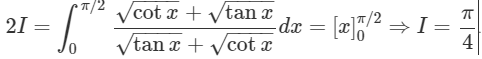Q.6. The area of the region bounded by the curve x² = 4y and the straight line x = 4y – 2 is
(a) ⅜ sq. units
(b) ⅝ sq. units
(c) ⅞ sq. units
(d) 9/8 sq. units

For the curves x2 = y and x = 4y-2, the points of intersection are x = -1 and x = 2.

Hence, the required area, A = -12 {[(x+2)/4]- [x2/4] } dx

Now, integrate the function and apply the limits, we get

A = (¼)[(10/3)-(-7/6)]

A = (¼)(9/2) = 9/8 sq. units

Hence, the correct answer is option (d) 9/8 sq. units

Q.7.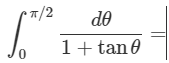(a) π|
(b) π/2|
(c) π/3|
(d) π/4|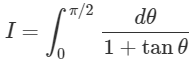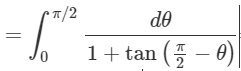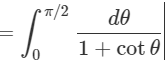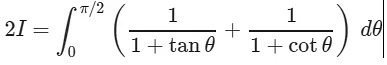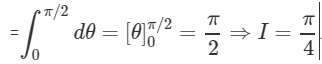Q.8. The area enclosed between the graph of y = xand the lines x = 0, y = 1, y = 8 is
(a) 7
(b) 14
(c) 45/6

(d) None of these

Given curve, y=x3 or x = y1/3.

Hence, the required area, A = 18 y1/3 dy

A = [(y4/3)/(4/3)]18

Now, apply the limits, we get

A = (¾)(16-1)

A = (¾)(15) = 45/4.

Hence, option (c) 45/4 is the correct answer.

Q.9.  if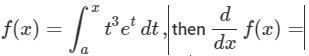(a) ex(x3+3x2
(b) x3ex
(c) a3ea
(d) None of these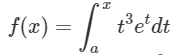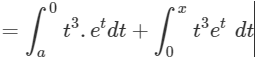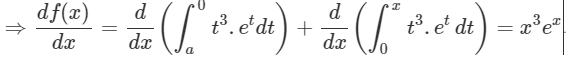Q.10.  The area of the region bounded by the curve y² = x, the y-axis and between y = 2 and y = 4 is
(a) 52/3 sq. units
(b) 54/3 sq. units
(c) 56/3 sq. units
(d) None of these

Given: y2 = x

Hence, the required area, A = 24 y2 dy

A = [y3/3]24

A = (43/3) – (23/3)

A = (64/3) – (8/3)

A = 56/3 sq. units.

Q.11.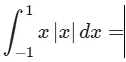(a) 1
(b) 0
(c) 2

(d) -2

Let f(x)=x|x|. Then f(−x)=−x|−x|=−x|x|=−f(x)
Therefore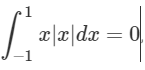(By the property of definite integral).

Q.12.  Area of the region bounded by the curve y = cos x between x = 0 and x = π is
(a) 1 sq. units
(b) 2 sq. units
(c)  3 sq. units
(d) 4 sq. units

Given: y= cos x and also provided that x= 0 and x = π

Hence, the required area, A =0π |cos x| dx

It can also be written as,

A = 20π/2 cos x dx

Now, integrate the function, we get

A = 2[sin x]0π/2

Now, apply the limits we get

A = 2 sq. units

Hence, the correct answer is option (b) 2 sq. units

Q.13.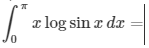(a)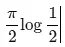(b)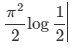(c)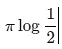(d)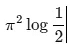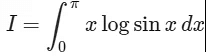...(i)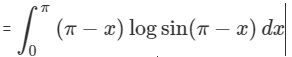....(ii)
By adding (i) and (ii), we get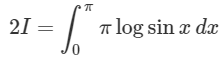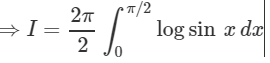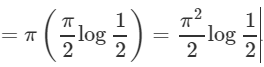Q.14.  Area of the region bounded by the curve x = 2y + 3, the y-axis and between y = -1 and y = 1 is
(a) 6 sq. units
(b) 4 sq. units
(c) 8 sq. units
(d) 3/2 sq. units

Required Area =-11(2y+3)dy

A=[(2y2/2)+3y]-11

Now, apply the limits, we get

A = 1+3-1+3

A = 6 sq. units.

Q.15.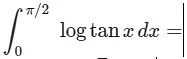(a)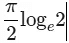(b)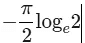(c)  πloge

(d) 0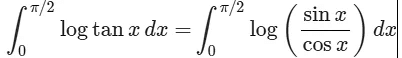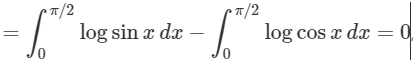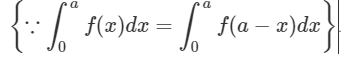Q.16. The area bounded by the curve y = x3, the x-axis and two ordinates x = 1 and x = 2 is
(a) 15/2 sq. units
(b) 15/4 sq. units
(c) 17/2 sq. units
(d) 17/4 sq. units

Required Area = 12 x3 dx

A = [x4/4]12
Now, apply the limits, we get
A = [(24/4) – (¼)]
A = (16/4) – (¼)
A = 15/4
Hence, the required area is 15/4 is the correct answer.

Q.17.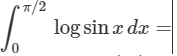(a)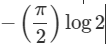(b)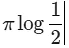(c)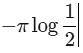(d)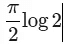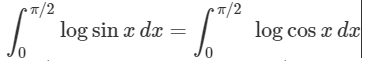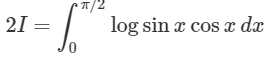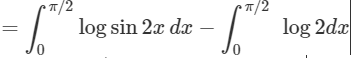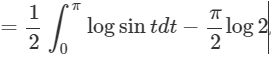(Putting 2x=t)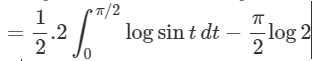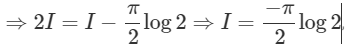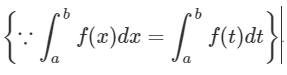Q.18. The area of the region bounded by the circle x² + y² = 1 is
(a) 2π sq. units
(b) 3π sq. units
(c) 4π sq. units
(d) 1π sq. units

Given:
Given circle equation is x2+y2 =1, whose centre is (0, 0) and radius is 1.
Therefore, y2 = 1-x2
y=√(1-x2)
Hence, the required area, A = 401√(1-x2) dx

Now, integrate the function and apply limits, we get
A = 4(½)(π/2)
A = π sq. units
Hence, option (d) 1π sq. unit is the correct answer.

Q.19.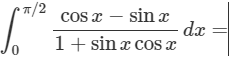(a) 2
(b) -2
(c) 0
(d) None of these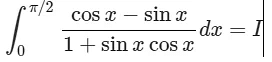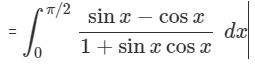Now,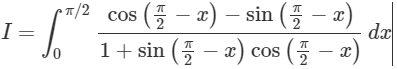On adding, 2I = 0 ⇒ I = 0.

Q.20. Area bounded by the curve y = sin x and the x-axis between x = 0 and x = 2π is
(a) 2 sq. units
(b) 3 sq. units
(c) 4 sq. units
(d) None of these

Required Area, A = 02π |sin x| dx
A = 0π sin x dx + π2π(-sin x) dx

Now, substitute the limits, we get

A= 4 sq. units.

The document Single Correct MCQs: Definite Integrals & Application of Integrals - Notes | Study All Types of Questions for JEE - JEE is a part of the JEE Course All Types of Questions for JEE.
All you need of JEE at this link: JEE

## All Types of Questions for JEE

376 docs
 Use Code STAYHOME200 and get INR 200 additional OFF

## All Types of Questions for JEE

376 docs

Track your progress, build streaks, highlight & save important lessons and more!

,

,

,

,

,

,

,

,

,

,

,

,

,

,

,

,

,

,

,

,

,

;